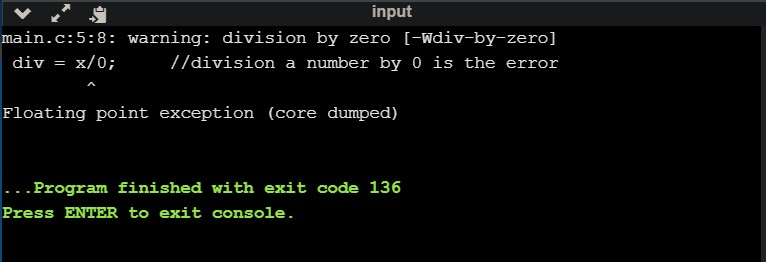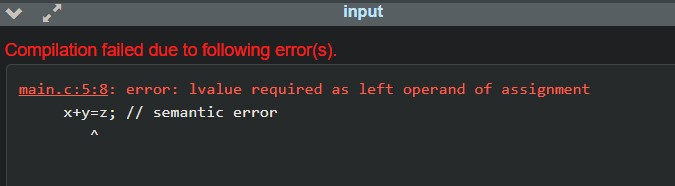## Types of Error in C

### What is error ?

When performing an operation is against the rule or the syntax of a code and as result of it, the program performs abnormal act then we can said that error occurred.
Programming errors often remain undetected until the program is executed or compiled. Thus, the errors must be removed for the desired output.

In this tutorial you will learn :

### Types of Error :

The types of error are classified into five categories in C.
1. Syntax error
2. Run time error
3. Logical error
5. Semantic error

### Syntax Error :

The errors that occur after violating the rules of writing C syntax are known as Syntax Errors.
Syntax errors are occurred at the compilation time that's why it is also known as Compilation
Errors
or Compile-time Errors. These errors can be easily corrected.

#### Some commonly occurred Syntax Errors are :

1. Missed the semicolon (;) at the end of any statement.
2. Missed the parenthesis ({}) while writing the code.
3. Using a variable without its declaration.

###### Example :
``#include<stdio.h> int main() {     Char m ; 	//The incorrect syntax of char     char n ;     printf("%c%c",m,n);     return 0; }``
The errors that occur during the execution-time even after successful compilation are known as Run-Time Errors. It generally occurs due to some illegal operation performed in the program. It's little bit difficult to find these types of errors as the compiler can't point to the line at which the error occurs.

#### Some commonly occurred Run time Errors are :

1. Deviding something by 0.
2. Lack of memory space.

###### Example :
``````#include <stdio.h>
int main()
{
int x = 9, div;
div = x/0;     //division a number by 0 is the error.
return 0;
}``````

##### Output :### Logical Error :

Errors which are error-free and syntactically correct but leads to an undesired output are known as the Logical Error. The occurrence of these errors mainly depend upon the logical thinking of the coder and are hard to detect as the programmer has to check the entire coding line by line.

#### Commonly occurred Logical Errors are :

1. Putting a semicolon (;) after the loop.
2. Incorrect translation of algorithm.
3. Poor understanding of the problem and a lack of clarity of hierarchy of operators.

###### Example
``````#include<stdio.h>
int main()
{
float a=117.56, b=117.57;
if(a==b)
printf("a and b are equal");
return 0;
}

// When a and b are float types value,
they rarely become equal due to truncation errors.
The printf call will not be executed at all.
``````
##### Output :The errors that occur when the executable file of the program is not created. These are errors generated when the executable of the program cannot be generated.

#### Some commonly occurred Linker Errors are :

1. Writing Main() instead of main().
2. Wrong function prototyping, incorrect header files.
###### Example :
``````#include<stdio.h>
int Main() // Here Main will be main
{
int x = 35;
printf("%d", x);
return 0;
}``````

### Semantic Error :

The errors that occur when the statements written in the program are not understandable by the compiler, are known as the Semantic Error. This is like the gramatical mistakes.

#### Some commonly occurred Syntax Errors are :

1. Array index out of bound.
``````  int f;
f=117;``````
2. Un-initialized variable.
``````  int a;
a=a+56;``````
3. Errors in expressions.
``````  int x, y, z;
x+y=z;``````

###### Example :
``````#include <stdio.h>
int main()
{
int x=117,y=56,z;
x+y=z; // semantic error
return 0;
}``````
##### Output :